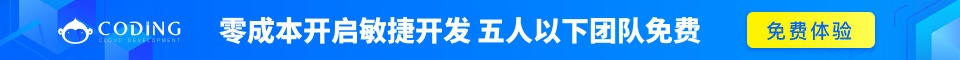document.cookie 将以字符串的方式返回所有的 cookies ，类型格式: cookie1=value; cookie2=value; cookie3=value;

{
var d = new Date();
d.setTime(d.getTime()+(exdays*24*60*60*1000));
var expires = "expires="+d.toGMTString();
document.cookie = cname + "=" + cvalue + "; " + expires;
}

{
var name = cname + "=";
for(var i=0; i<ca.length; i++)
{
var c = ca[i].trim();
if (c.indexOf(name)==0) return c.substring(name.length,c.length);
}
return "";
}

{
{
}
else
{
{
}
}
}

## 完整实例

### 实例

{
var d = new Date();
d.setTime(d.getTime()+(exdays*24*60*60*1000));
var expires = "expires="+d.toGMTString();
document.cookie = cname + "=" + cvalue + "; " + expires;
}

{
var name = cname + "=";
for(var i=0; i<ca.length; i++)
{
var c = ca[i].trim();
if (c.indexOf(name)==0) return c.substring(name.length,c.length);
}
return "";
}

{
if (user!="")
{
}
else
{